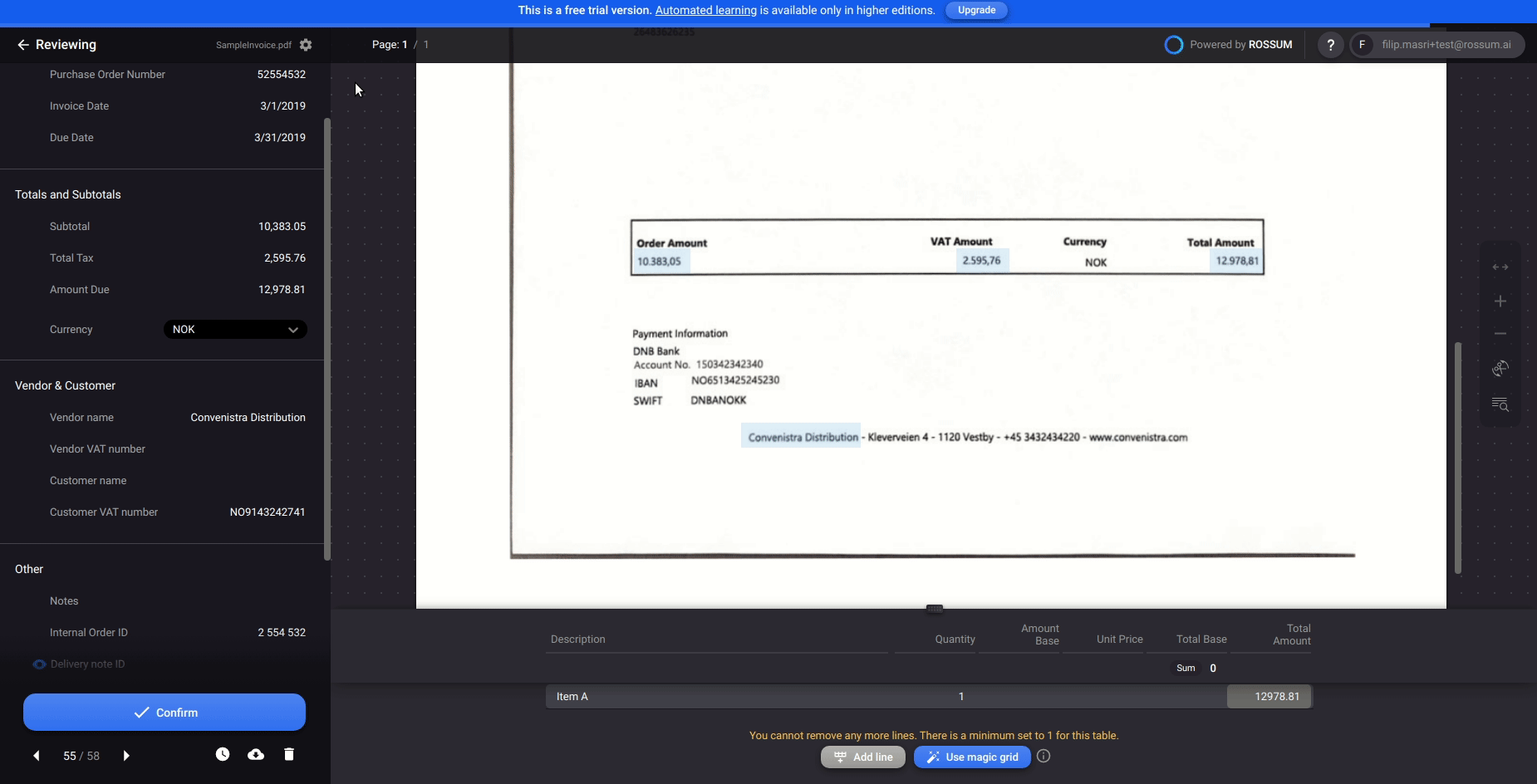# Amount due on document vs. sum of total amount column

Data integrity checks are a very common and powerful tool that can help operators capture data from documents faster. The most typical check is to compare the amount due on document and the sum of total amount line items column. If the two values do not match, you would like to show a message to the operator.

This code example assumes you have the following field IDs in your extraction schema:

• "amount_due" for capturing the total amount on the invoice
• "item_amount_total" for capturing the total amount with tax for each line item

Moreover, the serverless function should be configured to get notifications on "user_update" action and the function should be assigned to some queue.

``````// This serverless function example can be used for annotation_content events
// (e.g. user_update action). annotation_content events provide annotation
// content tree as the input.
//
// The function below shows how to compare the Amount Due on document
// to the sum of line items amount total. Warning message will be shown
// to the user if values do not match.

// --- ROSSUM HOOK REQUEST HANDLER ---

// The rossum_hook_request_handler is an obligatory main function that accepts
// input and produces output of the rossum serverless function hook. Currently,
// the only available programming languages are Javascript executed on Node.js 12 environment and Python.
// @param {Object} annotation - see https://api.elis.rossum.ai/docs/#annotation-content-event-data-format
// @returns {Object} - the messages and operations that update the annotation content

exports.rossum_hook_request_handler = ({
annotation: {
content
}
}) => {
try {

const [amountDueDatapoint] = findBySchemaId(
content,
'amount_due',
);

// List of all datapoints of item_amount_total schema id
const amountTotalColumnDatapoints = findBySchemaId(
content,
'item_amount_total',
);

// Getting the numberic value in normalized format
const amountDueString = amountDueDatapoint.content.normalized_value;

messages = [];

if(amountDueString && (amountTotalColumnDatapoints.length > 0)){

const amountDue = parseFloat(amountDueString);
const lineItemsSum = amountTotalColumnDatapoints.reduce(function (sum, datapoint) { return sum + parseFloat(datapoint.content.normalized_value)}, 0);

if(amountDue != lineItemsSum){
messages.push(
createMessage(
'warning',
`Total amount on document (\${amountDue}) does not match the sum of line items total amount (\${lineItemsSum})`
)
);
}
}

// Return messages and operations to be used to update current annotation data
return {
messages
};
} catch (e) {
// In case of exception, create and return error message. This may be useful for debugging.
const messages = [
createMessage('error', 'Serverless Function: ' + e.message)
];
return {
messages,
};
}
};

// --- HELPER FUNCTIONS ---

// Return datapoints matching a schema id.
// @param {Object} content - the annotation content tree (see https://api.elis.rossum.ai/docs/#annotation-data)
// @param {string} schemaId - the field's ID as defined in the extraction schema(see https://api.elis.rossum.ai/docs/#document-schema)
// @returns {Array} - the list of datapoints matching the schema ID

const findBySchemaId = (content, schemaId) =>
content.reduce(
(results, dp) =>
dp.schema_id === schemaId ?
[...results, dp] :
dp.children ?
[...results, ...findBySchemaId(dp.children, schemaId)] :
results,
[],
);

// Create a message which will be shown to the user
// @param {number} datapointId - the id of the datapoint where the message will appear (null for "global" messages).
// @param {String} messageType - the type of the message, any of {info|warning|error}. Errors prevent confirmation in the UI.
// @param {String} messageContent - the message shown to the user
// @returns {Object} - the JSON message definition (see https://api.elis.rossum.ai/docs/#annotation-content-event-response-format)

const createMessage = (type, content, datapointId = null) => ({
content: content,
type: type,
id: datapointId,
});

// Replace the value of the datapoint with a new value.
// @param {Object} datapoint - the content of the datapoint
// @param {string} - the new value of the datapoint
// @return {Object} - the JSON replace operation definition (see https://api.elis.rossum.ai/docs/#annotation-content-event-response-format)

const createReplaceOperation = (datapoint, newValue) => ({
op: 'replace',
id: datapoint.id,
value: {
content: {
value: newValue,
},
},
});
``````